# Search by Topic

Filter by: Content type:
Age range:
Challenge level:

### There are 36 results

Broad Topics > Numbers and the Number System > Square numbers### Always, Sometimes or Never? Number

##### Age 7 to 11 Challenge Level:

Are these statements always true, sometimes true or never true?### Light the Lights Again

##### Age 7 to 11 Challenge Level:

Each light in this interactivity turns on according to a rule. What happens when you enter different numbers? Can you find the smallest number that lights up all four lights?### Picture a Pyramid ...

##### Age 7 to 11 Challenge Level:

Imagine a pyramid which is built in square layers of small cubes. If we number the cubes from the top, starting with 1, can you picture which cubes are directly below this first cube?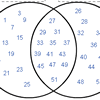### Various Venns

##### Age 7 to 11 Challenge Level:

Use the interactivities to complete these Venn diagrams.### Factors and Multiples Puzzle

##### Age 11 to 14 Challenge Level:

Using your knowledge of the properties of numbers, can you fill all the squares on the board?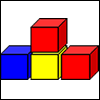### Up and Down Staircases

##### Age 7 to 11 Challenge Level:

One block is needed to make an up-and-down staircase, with one step up and one step down. How many blocks would be needed to build an up-and-down staircase with 5 steps up and 5 steps down?### Odd Squares

##### Age 7 to 11 Challenge Level:

Think of a number, square it and subtract your starting number. Is the number you’re left with odd or even? How do the images help to explain this?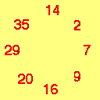### Cycling Squares

##### Age 7 to 11 Challenge Level:

Can you make a cycle of pairs that add to make a square number using all the numbers in the box below, once and once only?### Two Primes Make One Square

##### Age 7 to 11 Challenge Level:

Can you make square numbers by adding two prime numbers together?### One Wasn't Square

##### Age 7 to 11 Challenge Level:

Mrs Morgan, the class's teacher, pinned numbers onto the backs of three children. Use the information to find out what the three numbers were.### Fractions in a Box

##### Age 7 to 11 Challenge Level:

The discs for this game are kept in a flat square box with a square hole for each. Use the information to find out how many discs of each colour there are in the box.### Iff

##### Age 14 to 18 Challenge Level:

Take a triangular number, multiply it by 8 and add 1. What is special about your answer? Can you prove it?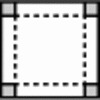### Making Boxes

##### Age 7 to 11 Challenge Level:

Cut differently-sized square corners from a square piece of paper to make boxes without lids. Do they all have the same volume?### Swimming Pool Tiles

##### Age 7 to 11 Challenge Level:

This activity creates an opportunity to explore all kinds of number-related patterns.### Filling the Gaps

##### Age 14 to 16 Challenge Level:

Which numbers can we write as a sum of square numbers?### Few and Far Between?

##### Age 14 to 18 Challenge Level:

Can you find some Pythagorean Triples where the two smaller numbers differ by 1?### Generating Triples

##### Age 14 to 16 Challenge Level:

Sets of integers like 3, 4, 5 are called Pythagorean Triples, because they could be the lengths of the sides of a right-angled triangle. Can you find any more?### Robert's Spreadsheet

##### Age 14 to 16 Challenge Level:

Robert noticed some interesting patterns when he highlighted square numbers in a spreadsheet. Can you prove that the patterns will continue?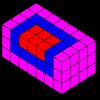### Summing Squares

##### Age 14 to 16 Challenge Level:

Discover a way to sum square numbers by building cuboids from small cubes. Can you picture how the sequence will grow?### Sticky Numbers

##### Age 11 to 14 Challenge Level:

Can you arrange the numbers 1 to 17 in a row so that each adjacent pair adds up to a square number?### Numbers as Shapes

##### Age 5 to 7 Challenge Level:

Use cubes to continue making the numbers from 7 to 20. Are they sticks, rectangles or squares?### Triangles Within Squares

##### Age 14 to 16 Challenge Level:

Can you find a rule which relates triangular numbers to square numbers?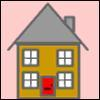### Smith and Jones

##### Age 14 to 16 Challenge Level:

Mr Smith and Mr Jones are two maths teachers. By asking questions, the answers to which may be right or wrong, Mr Jones is able to find the number of the house Mr Smith lives in... Or not!### A Square Deal

##### Age 7 to 11 Challenge Level:

Complete the magic square using the numbers 1 to 25 once each. Each row, column and diagonal adds up to 65.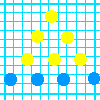### Graphing Number Patterns

##### Age 7 to 11 Challenge Level:

Does a graph of the triangular numbers cross a graph of the six times table? If so, where? Will a graph of the square numbers cross the times table too?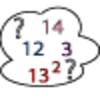### Seven Square Numbers

##### Age 7 to 11 Challenge Level:

Add the sum of the squares of four numbers between 10 and 20 to the sum of the squares of three numbers less than 6 to make the square of another, larger, number.### Augustus' Age

##### Age 7 to 11 Challenge Level:

In 1871 a mathematician called Augustus De Morgan died. De Morgan made a puzzling statement about his age. Can you discover which year De Morgan was born in?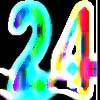### Special 24

##### Age 7 to 11 Challenge Level:

Find another number that is one short of a square number and when you double it and add 1, the result is also a square number.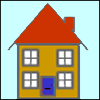### A Right Charlie

##### Age 7 to 11 Challenge Level:

Can you use this information to work out Charlie's house number?### Triangular Triples

##### Age 14 to 16 Challenge Level:

Show that 8778, 10296 and 13530 are three triangular numbers and that they form a Pythagorean triple.### Digat

##### Age 11 to 14 Challenge Level:

What is the value of the digit A in the sum below: [3(230 + A)]^2 = 49280A### Square Routes

##### Age 11 to 14 Challenge Level:

How many four digit square numbers are composed of even numerals? What four digit square numbers can be reversed and become the square of another number?### Time of Birth

##### Age 11 to 14 Challenge Level:

A woman was born in a year that was a square number, lived a square number of years and died in a year that was also a square number. When was she born?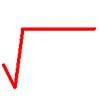### The Root Cause

##### Age 16 to 18 Challenge Level:

Prove that if a is a natural number and the square root of a is rational, then it is a square number (an integer n^2 for some integer n.)### Little Squares

##### Age 5 to 7 Challenge Level:

Look at the squares in this problem. What does the next square look like? I draw a square with 81 little squares inside it. How long and how wide is my square?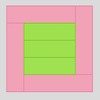### Cuisenaire Rods

##### Age 7 to 11 Challenge Level:

These squares have been made from Cuisenaire rods. Can you describe the pattern? What would the next square look like?